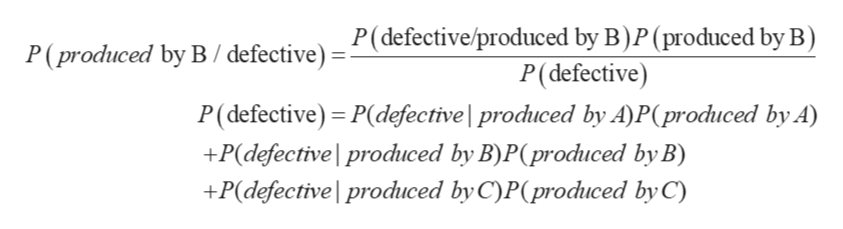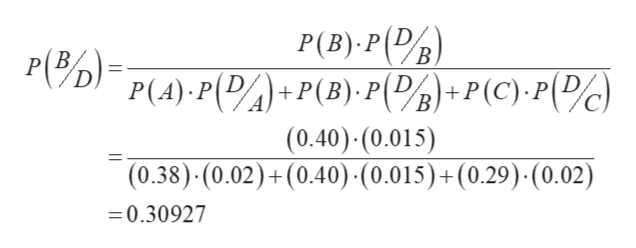# In a manufacturing plant, three machines, A, B, and C, produce 38%, 33%, and 29%, respectively, of the total production. The company's quality-control department has determined that 2% of the items produced by machine A, 1.5% of the items produced by machine B, and 2% of the items produced by machine C are defective. If an item is selected at random and found to be defective, what is the probability that it was produced by machine B? (Round your answer to three decimal places.)

Question

In a manufacturing plant, three machines, A, B, and C, produce 38%, 33%, and 29%, respectively, of the total production. The company's quality-control department has determined that 2% of the items produced by machine A, 1.5% of the items produced by machine B, and 2% of the items produced by machine C are defective. If an item is selected at random and found to be defective, what is the probability that it was produced by machine B? (Round your answer to three decimal places.)

check_circleExpert Solution
Step 1

Machine A, B, C produces 38%, 33%, and 29%.

P(A) = 0.38 %, P (B) = 0.40 and P(C) = 0.29%

D denote the defective item.

2% of the items produced by machine A are defective, so P(D/A) = 0.02

1.5% of the items produced by machine B are defective, so P(D/B) = 0.015

2% of the items produced by machine C are defective, so P(D/C) = 0.02

To find the probability which is produced by machine B, or P(B/D).

Now from the Bayes’ Theorem:help_outlineImage TranscriptioncloseP(defective/produced by B)P (produced by B) '(produced by B/defective) P(defective) P(defective) - P(defective | produced by A)P(produced by A) +P(defective produced by B)P(produced by B) +P(defective produced by C)P(produced by C) fullscreen
Step 2

put the given values i...help_outlineImage TranscriptioncloseP(B) P DB В, P(D P(4) P(DA)+P(B).PD/*P(C)P(c) (0.40)-(0.015) (0.38) (0.02)+(0.40) -(0.015) + (0.29) (0.02) -0.30927 fullscreen

### Want to see the full answer?

See Solution

#### Want to see this answer and more?

Solutions are written by subject experts who are available 24/7. Questions are typically answered within 1 hour*

See Solution
*Response times may vary by subject and question
Tagged in

### Math